# Transforming rows: row-by-row processing of tables

All the C# code examples presented on this page can be found in the related demo function of MS Visual Studio project FinaquantProtosStarter which can be downloaded at the product page of the free .NET library finaquant® protos.
Even though quite easy to understand and use, TransformRowsDic()1 in the free .net library finaquant® protos is one of the most versatile and useful table transformation functions. This table function offers a simple way for applying user-defined formulas on each row of a table.

Using this row transformer you can:

1. derive the value of a field of a table in terms of other related fields.
2. apply logical and mathematical operations on some fields of table.

Following examples illustrate how TransformRowsDic() can be used in practice for the purposes listed above. All the examples start with the TestTable below as the primary input parameter2.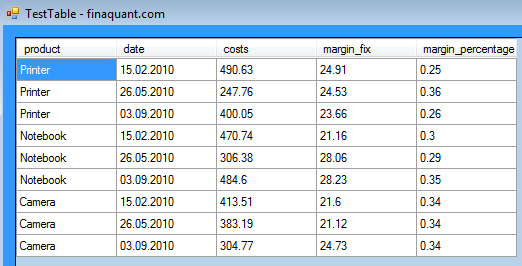## Example 1: Calculating related key figure “price” for each row

This example illustrates how the values of a new key figure price is assigned with the user defined formula:

`price = (1 + margin_percentage) x costs`
```// see user-defined function UserFunctions.CalculatePriceDic_1() // KeyFigDic["price"] = (1 + KeyFigDic["margin_percentage"]) * KeyFigDic["costs"];   // insert new key figure "price" into table OutputTbl = MatrixTable.InsertNewColumn(TestTable, "price", 0.0); // transform rows OutputTbl = MatrixTable.TransformRowsDic(OutputTbl, UserFunctions.CalculatePriceDic_1); // view output table MatrixTable.View_MatrixTable(OutputTbl, "CASE 1: price = (1 + margin_percentage) x costs");```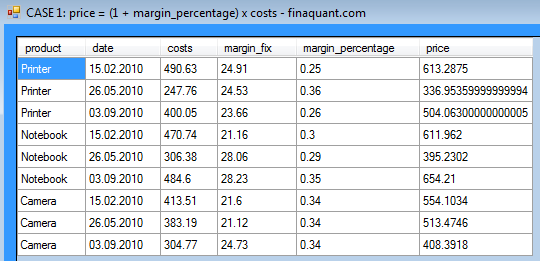User-defined function CalculatePriceDic_1() as delegate function input to row transformer TransformRowsDic:

```// User-defined function for transforming rows (row-by-row processing) // price = (1 + margin_percentage) * costs public static void CalculatePriceDic_1( ref Dictionary<string, string> TextAttribDic, ref Dictionary<string, int> NumAttribDic, ref Dictionary<string, double> KeyFigDic) { // user-defined formula for price KeyFigDic["price"] = (1 + KeyFigDic["margin_percentage"]) * KeyFigDic["costs"]; }```

Though it may seem complicated at first sight, the whole user-defined function above is nothing other than an envelope for the formula:

`KeyFigDic["price"] = (1 + KeyFigDic["margin_percentage"]) * KeyFigDic["costs"];`

## Example 2: Calculating “price” with conditional logic

In this example, the formula to be applied for calculating price depends on product:

```price = (1 + margin_percentage) x costs, for products "Printer" and "Notebook"
price = margin_fix + costs, for product "Camera"
```
```// insert new key figure "price" into table OutputTbl = MatrixTable.InsertNewColumn(TestTable, "price", 0.0); // transform rows OutputTbl = MatrixTable.TransformRowsDic(OutputTbl, UserFunctions.CalculatePriceDic_2); // view output table MatrixTable.View_MatrixTable(OutputTbl, "CASE 2: Percentage price margin for \"Printer\" and \"Notebook\", additive margin for \"Camera\"");```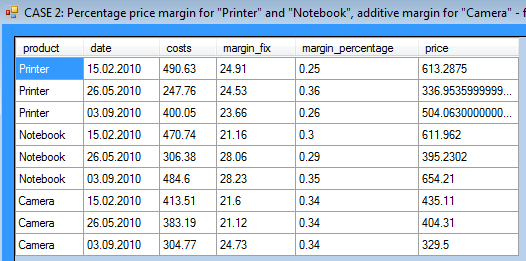User defined function CalculatePriceDic_2() with conditional logic:

```// - price = (1 + margin_percentage) x costs, for products "Printer" and "Notebook" // - price = margin_fix + costs, for product "Camera" // Note: price depends on some other key figures and text attribute "product" public static void CalculatePriceDic_2( ref Dictionary<string, string> TextAttribDic, ref Dictionary<string, int> NumAttribDic, ref Dictionary<string, double> KeyFigDic) { // user-defined conditional formula for price if (TextAttribDic["product"] == "Printer" || TextAttribDic["product"] == "Notebook") KeyFigDic["price"] = (1 + KeyFigDic["margin_percentage"]) * KeyFigDic["costs"];   if (TextAttribDic["product"] == "Camera") KeyFigDic["price"] = KeyFigDic["margin_fix"] + KeyFigDic["costs"]; }```

Note that the condition logic (i.e. which formula is to be applied for which products) is hard-coded in the example above. The following example shows, how the same problem could be solved in a more elegant way, by defining the margin type (fix or percentage) for each product as a table parameter.

## Example 3: Calculating “price” with a table parameter containing margin type

Beside implementing a more parametric and flexible approach compared to the second example above, this example also illustrates the practical use of combining tables in order to gather all relevant parameters in a single table.

A margin type for each product is defined in MarginTypeTable: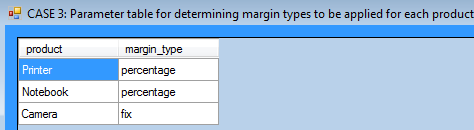```// combine TestTable and MarginTypeTable OutputTbl = MatrixTable.CombineTablesFirstMatch(TestTable, MarginTypeTable); // view combined table MatrixTable.View_MatrixTable(OutputTbl, "CASE 3: Combined table");```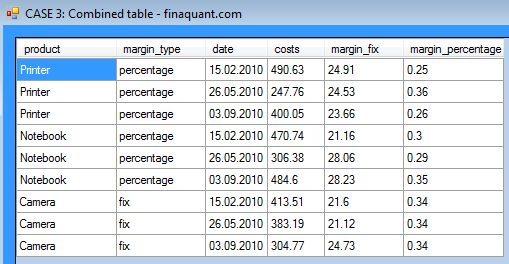Now that we have all the relevant parameters including margin type in a single combined table, conditional formula for price can be applied on each row of table:

```// insert new key figure "price" into table OutputTbl = MatrixTable.InsertNewColumn(OutputTbl, "price", 0.0); // transform rows OutputTbl = MatrixTable.TransformRowsDic(OutputTbl, UserFunctions.CalculatePriceDic_3); // view output table MatrixTable.View_MatrixTable(OutputTbl, "CASE 3: Price calculation with MarginTypeTable for determining margin types to be applied");```Written by: Tunç Ali Kütükçüoglu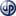Copyright secured by Digiprove © 2013 Tunc Ali Kütükcüoglu
in the free .net library finaquant® protos is one of the most versatile and useful table transformation functions. This table" data-image="https://software.tuncalik.com/wp-content/uploads/2013/01/RowTransformer_TestTable.png" data-button="">1. Transform rows of a table using dictionaries as associative arrays for keeping field names and values. []
2. These examples can be found as demo function in the Visual Studio project “FinaquantProtosStarter” which can be downloaded at the product page of finaquant® protos, in versions 1.02 and higher. []
3. Transform rows of a table using dictionaries as associative arrays for keeping field names and values. []
This entry was posted in Calculation engine and tagged , , . Bookmark the permalink.